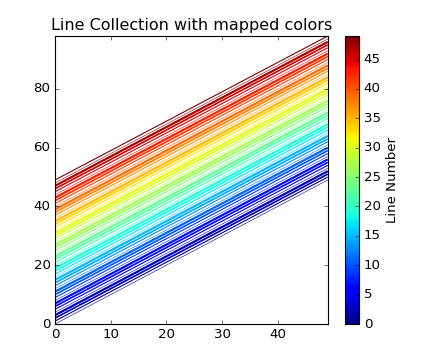### We're updating the default styles for Matplotlib 2.0

Learn what to expect in the new updatesTravis-CI:# pylab_examples example code: line_collection2.py¶```import matplotlib.pyplot as plt
import numpy as np
from matplotlib.collections import LineCollection

# In order to efficiently plot many lines in a single set of axes,
# Matplotlib has the ability to add the lines all at once. Here is a
# simple example showing how it is done.

N = 50
x = np.arange(N)
# Here are many sets of y to plot vs x
ys = [x + i for i in x]

# We need to set the plot limits, they will not autoscale
ax = plt.axes()
ax.set_xlim((np.amin(x), np.amax(x)))
ax.set_ylim((np.amin(np.amin(ys)), np.amax(np.amax(ys))))

# colors is sequence of rgba tuples
# linestyle is a string or dash tuple. Legal string values are
#          solid|dashed|dashdot|dotted.  The dash tuple is (offset, onoffseq)
#          where onoffseq is an even length tuple of on and off ink in points.
#          If linestyle is omitted, 'solid' is used

# Make a sequence of x,y pairs
line_segments = LineCollection([list(zip(x, y)) for y in ys],
linewidths=(0.5, 1, 1.5, 2),
linestyles='solid')
line_segments.set_array(x)